Test: Time Domain Analysis of Control Systems- 2

# Test: Time Domain Analysis of Control Systems- 2 - Electrical Engineering (EE)

Test Description

## 20 Questions MCQ Test GATE Electrical Engineering (EE) 2024 Mock Test Series - Test: Time Domain Analysis of Control Systems- 2

Test: Time Domain Analysis of Control Systems- 2 for Electrical Engineering (EE) 2023 is part of GATE Electrical Engineering (EE) 2024 Mock Test Series preparation. The Test: Time Domain Analysis of Control Systems- 2 questions and answers have been prepared according to the Electrical Engineering (EE) exam syllabus.The Test: Time Domain Analysis of Control Systems- 2 MCQs are made for Electrical Engineering (EE) 2023 Exam. Find important definitions, questions, notes, meanings, examples, exercises, MCQs and online tests for Test: Time Domain Analysis of Control Systems- 2 below.
Solutions of Test: Time Domain Analysis of Control Systems- 2 questions in English are available as part of our GATE Electrical Engineering (EE) 2024 Mock Test Series for Electrical Engineering (EE) & Test: Time Domain Analysis of Control Systems- 2 solutions in Hindi for GATE Electrical Engineering (EE) 2024 Mock Test Series course. Download more important topics, notes, lectures and mock test series for Electrical Engineering (EE) Exam by signing up for free. Attempt Test: Time Domain Analysis of Control Systems- 2 | 20 questions in 60 minutes | Mock test for Electrical Engineering (EE) preparation | Free important questions MCQ to study GATE Electrical Engineering (EE) 2024 Mock Test Series for Electrical Engineering (EE) Exam | Download free PDF with solutions
 1 Crore+ students have signed up on EduRev. Have you?
Test: Time Domain Analysis of Control Systems- 2 - Question 1

### The system shown in figure below has unit step input.In order that the steady state error is 0.1, the value of K required should be

Detailed Solution for Test: Time Domain Analysis of Control Systems- 2 - Question 1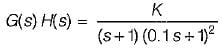Since Input is unit step, therefore steady state error is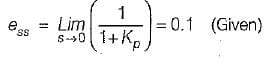Here,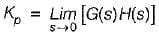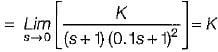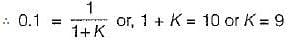Test: Time Domain Analysis of Control Systems- 2 - Question 2

### The steady-state error coefficients for a system are Kp = ∞ , Kv = finite constant and Ka = 0. The type of the system is

Detailed Solution for Test: Time Domain Analysis of Control Systems- 2 - Question 2

We have,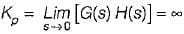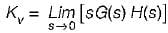= finite constant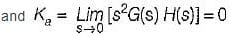From above values of Kp, Kv and Ka we conclude that the type of the system should be one.

Test: Time Domain Analysis of Control Systems- 2 - Question 3

### The steady-state error of a feedback control system with an acceleration input becomes finite in a

Detailed Solution for Test: Time Domain Analysis of Control Systems- 2 - Question 3

Steady state error with an acceleration input having an amplitude of A is given by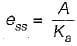where,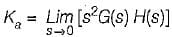Hence, if the type of the system = 2, then Ka = some non-zero value or finite value due to which we will get some finite vaiue of Ka.

Test: Time Domain Analysis of Control Systems- 2 - Question 4

Consider the unity feedback system shown below:​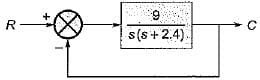The settling time of the resulting second order system for 2% tolerance band will be

Detailed Solution for Test: Time Domain Analysis of Control Systems- 2 - Question 4

The characteristic equation of the given closed loop system is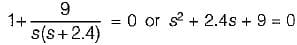Comparing above equation with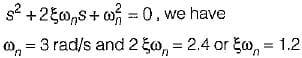Thus, setting time for 2% tolerance band is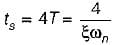or,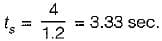Test: Time Domain Analysis of Control Systems- 2 - Question 5

For a control system, the Laplace transform of error signal e(t) is given by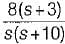. The steady state value of the error will be

Detailed Solution for Test: Time Domain Analysis of Control Systems- 2 - Question 5

Given,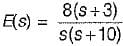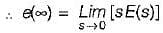(Using final value theorem)
or,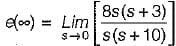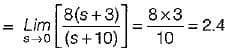Test: Time Domain Analysis of Control Systems- 2 - Question 6

For a type one system, the steady-state error due to step input is equal to

Detailed Solution for Test: Time Domain Analysis of Control Systems- 2 - Question 6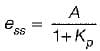where,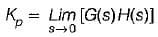(Here, A = magnitude of step input)
Since system is type -1, therefore Kp = ∞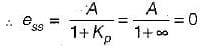Test: Time Domain Analysis of Control Systems- 2 - Question 7

For an unity feedback control system withthe value of K for damping ratio of 0.5 is

Detailed Solution for Test: Time Domain Analysis of Control Systems- 2 - Question 7

Characteristic equation is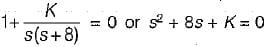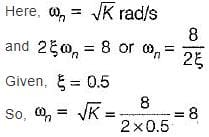or, K = 64

Test: Time Domain Analysis of Control Systems- 2 - Question 8

The damping ratio of a system having the characteristic equation s2 + 2s + 8 = 0 is

Detailed Solution for Test: Time Domain Analysis of Control Systems- 2 - Question 8

Given, s2 + 2s + 8 = 0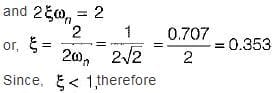system is underdamped.

Test: Time Domain Analysis of Control Systems- 2 - Question 9

The closed-loop transfer function of a unity - feedback system is given by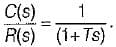The steady state error to a unit ramp input is:

Detailed Solution for Test: Time Domain Analysis of Control Systems- 2 - Question 9

Given,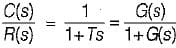(Since H(s) = 1)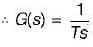For a unit ramp input,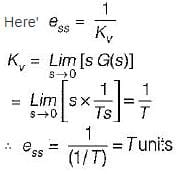Test: Time Domain Analysis of Control Systems- 2 - Question 10

The peak overshoot of step-input response of an underdamped second-order system is an indication of

Detailed Solution for Test: Time Domain Analysis of Control Systems- 2 - Question 10

If damping of system increases, peak overshoot Mp decreases and vice-versa.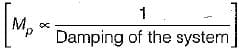Test: Time Domain Analysis of Control Systems- 2 - Question 11

Consider the position control system shown below: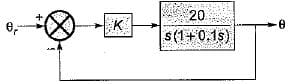The value of K such that the steady state error is 20° for input θr = 300t rad/sec, is

Detailed Solution for Test: Time Domain Analysis of Control Systems- 2 - Question 11

Input = 300t rad/sec = ramp input of magnitude A = 300.
For ramp input, steady state error is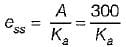where,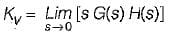Now, from given block diagram, we have: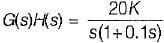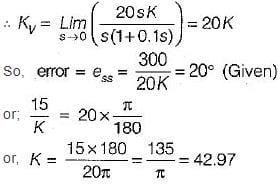So, value of gain is K = 42.97

Test: Time Domain Analysis of Control Systems- 2 - Question 12

Assertion (A): With the increase in bandwidth of the system the response of the system becomes fast.
Reason (R): Damping ratio of the system decreases with the increase in bandwidth.

Detailed Solution for Test: Time Domain Analysis of Control Systems- 2 - Question 12

When BW is increased, the system response becomes fast due to fait in rise time (tr).
With the increase in bandwidth of the system, damping ratio (ξ) decreases.
Thus, both assertion and reason are true but, reason is not the correct explanation of assertion.

Test: Time Domain Analysis of Control Systems- 2 - Question 13

For the stable system described by the block diagram shown below, Match List - I (Static error coefficients) with List - II (Values) and select the correct answer using the codes given below the lists: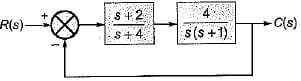List-I
A. Ka
B. Kv
C. Kp

List-II
1. ∞
2. 0
3. 2
4. -1

Codes:Detailed Solution for Test: Time Domain Analysis of Control Systems- 2 - Question 13

We have,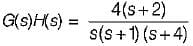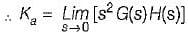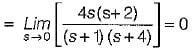Also,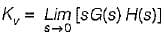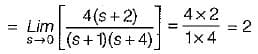and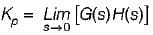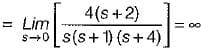Test: Time Domain Analysis of Control Systems- 2 - Question 14

The unit step response of a system is given by c(t) - 1 + 0.25 e-50t - 1.25 e-10t
The given system is

Detailed Solution for Test: Time Domain Analysis of Control Systems- 2 - Question 14

Given,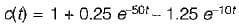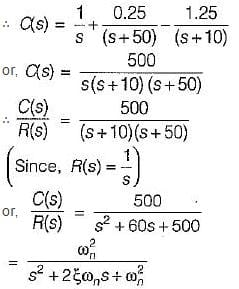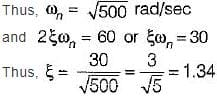Since, ξ > 1, therefore given system is overdamped.

Test: Time Domain Analysis of Control Systems- 2 - Question 15

Match List - I (Transfer function of systems) with List - II (Nature of damping) and select the correct answer using the codes given below the lists: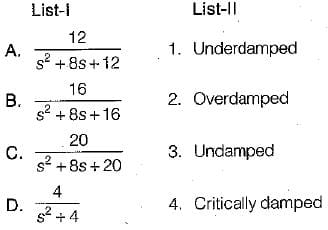Codes: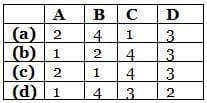Detailed Solution for Test: Time Domain Analysis of Control Systems- 2 - Question 15

Characteristic equations are:
• s2 + 8s + 12 = 0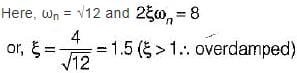• s2 + 8s + 16 = 0
Here, ωn = 4 and 2 ξωn = 8
or, ξ = 1 (critically damped)
• s2 + 8s + 20 = 0
Here, ω = √20
and 2ξω= 8
or, ξ = 0.894 (ξ < 1 ∴ underdamped)
• s2 + 4 = 0
or, ωn = 2
and ξ = 0 (∴ undamped)

Test: Time Domain Analysis of Control Systems- 2 - Question 16

The block diagram of an electronic pacemaker is given in figure below.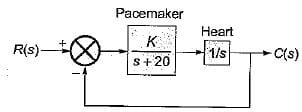What is the value of K for which the steady-state error to a unit ramp input is 0.02?

Detailed Solution for Test: Time Domain Analysis of Control Systems- 2 - Question 16

Here,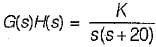As the input is a unit ramp function, therefore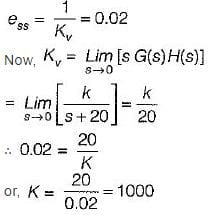Test: Time Domain Analysis of Control Systems- 2 - Question 17

Which one of the following equations gives the steady-state error for a unity feedback system excited by r(t) = 2 + 5t + 2t2 ?

Detailed Solution for Test: Time Domain Analysis of Control Systems- 2 - Question 17

Given, r(t) = 2 + 5t + 2t2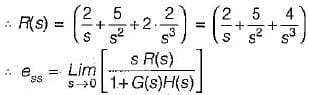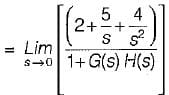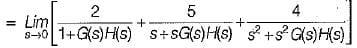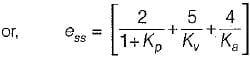Test: Time Domain Analysis of Control Systems- 2 - Question 18

An unity feedback control system with closed loon transfer function is given by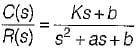The steady state error due a unit ramp input response is

Detailed Solution for Test: Time Domain Analysis of Control Systems- 2 - Question 18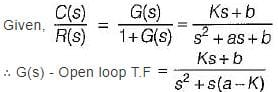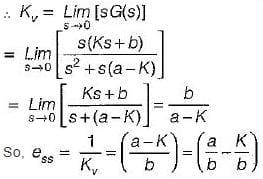Test: Time Domain Analysis of Control Systems- 2 - Question 19

The second order approximation using dominant pole concept for the transfer function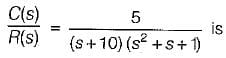Detailed Solution for Test: Time Domain Analysis of Control Systems- 2 - Question 19

Given,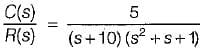In time constant form,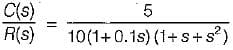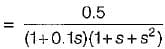Using dominant pole concept, the given transfer function can be approximated to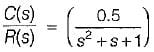Test: Time Domain Analysis of Control Systems- 2 - Question 20

The unit step response of the system represented by the block diagram shown below is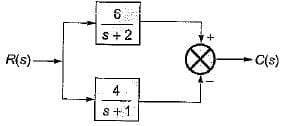Detailed Solution for Test: Time Domain Analysis of Control Systems- 2 - Question 20

From given block diagram, we have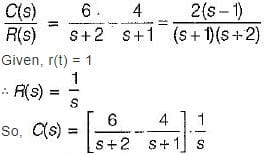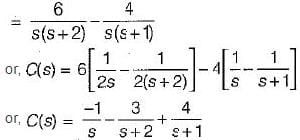∴ c(t) = (4e-t - 3e-2t - 1)
= Required step response

## GATE Electrical Engineering (EE) 2024 Mock Test Series

23 docs|285 tests
Information about Test: Time Domain Analysis of Control Systems- 2 Page
In this test you can find the Exam questions for Test: Time Domain Analysis of Control Systems- 2 solved & explained in the simplest way possible. Besides giving Questions and answers for Test: Time Domain Analysis of Control Systems- 2, EduRev gives you an ample number of Online tests for practice

## GATE Electrical Engineering (EE) 2024 Mock Test Series

23 docs|285 tests